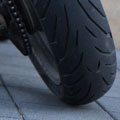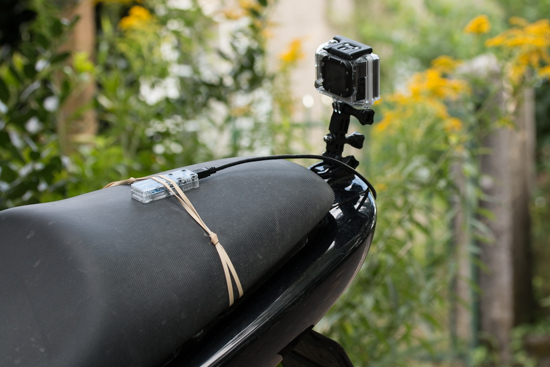Record the lean angle of a motorbike

# Record the lean angle of a motorbikeIn a motorbike race, the most spectacular is the way drivers lean their motorbikes in curves. Television channels often broadcast videos of on-board cameras and inlay the highest angle reached. For Grand Prix, this information is retrieved by telemetry and broadcasted in real time. But is it possible to produce a video of the same type (but not in real time) using a Yocto-3D and a GoPro?

To produce a video of this type, you must be able to register the video and the position of the motorbike. To register the video, we used a GoPro camera fixed a the rear of the motorbike. To register the motorbike position, we used a Yocto-3D powered by a USB battery. The Yocto-3D can be fixed anywhere on the bike, as long as it is in the axis of the bike and horizontal (we could put the Yocto-3D in any direction, but this would render angle computations much more complex as the initial position must be taken into account). To keep the system readily available, we put the Yocto-3D next to the GoPro camera.The GoPro, the Yocto-3D, and the battery assembled on the motorbike saddle spoiler

To inlay the bike angle on the video, we wrote a short C# program. We chose C# because we used the AForge library, but it is quite possible to write an equivalent program with another language or another video library. This piece of code has two functions. First, to configure the Yocto-3D so that it start registering data as soon as it is powered. Second, to retrieve the data registered on the Yocto-3D flash memory to inlay them on the GoPro video.The graph is inlayed on the video thanks to the AForge C# library

By default, the Yocto-3D doesn't register any measure in its flash memory. To use the Yocto-3D as a recorder and for our program to be able to use the data, we must:

1. Delete all the previously registered data
2. Define the recording frequency for the 4 quaternion functions to 30 measures per second
3. Set the autoStart function of the data logger so that the Yocto-3D starts recording as soon as it is powered
4. Save this configuration in the module flash memory (to prevent losing this configuration when the module is disconnected next)

private void ConfigureYocto3d(string serial)
{
YDataLogger logger = YDataLogger.FindDataLogger(serial + ".dataLogger");
logger.set_recording(YDataLogger.RECORDING_OFF);
logger.forgetAllDataStreams();
YQt q1 = YQt.FindQt(serial + ".qt1");
q1.set_logFrequency("30/s");
YQt q2 = YQt.FindQt(serial + ".qt2");
q2.set_logFrequency("30/s");
YQt q3 = YQt.FindQt(serial + ".qt3");
q3.set_logFrequency("30/s");
YQt q4 = YQt.FindQt(serial + ".qt4");
q4.set_logFrequency("30/s");
logger.set_autoStart(YDataLogger.AUTOSTART_ON);
logger.get_module().saveToFlash();
}

It is very important to run this function before you go for a drive. Otherwise, you may find data from a preceding recording or your Yocto-3D may not record the correct information. It is also important to connect the Yocto-3D at the same time that your start recording the video for both to be synchronized.

### Quaternions

It is tempting to use the tilt1 function to compute the motorbike angle. However, this function uses acceleration to compute the module tilt (see the module presentation). This measure is perturbed when the motorbike accelerates or decelerates. Moreover, this measure is highly averaged to obtain a great precision and is refreshed only 10 times per second. The best solution is to use the gyroscope (which is not perturbed by the supported accelerations) to compute the motorbike angle.

The three angles describing the module position in space (roll, heading, and pitch) are computed using the four components of the hyper-complex quaternion describing the module position. Normally, you don't have to worry about what a quaternion is because the API performs all the computations for you. You only need to register a callback function (with the registerAnglesCallback function) to receive the roll, heading and pitch angles as soon as you move the Yocto-3D.

Unfortunately, the data logger cannot directly register the three angles describing the module orientation. It can however store the four quaternions allowing you to recompute these three angles. Thus, to determine the motorbike angle in a curve, the data logger must register the four YQt functions of the Yocto-3D and recompute the angle with the following piece of code:

List<double> ComputeAngles(double w, double x, double y, double z, double defaultRoll)
{
double sqw = 0;
double sqx = 0;
double sqy = 0;
double sqz = 0;
double norm = 0;
double delta = 0;
double pitch = 0;
double roll = 0;
List<double> res = new List<double>();
sqw = w * w;
sqx = x * x;
sqy = y * y;
sqz = z * z;
norm = sqx + sqy + sqz + sqw;
delta = y * w - x * z;
if (delta > 0.499 * norm)
{
roll = defaultRoll;
pitch = 90.0;
head = Math.Round(2.0 * 1800.0 / Math.PI * Math.Atan2(x, w)) / 10.0;
}
else
{
if (delta < -0.499 * norm)
{
roll = defaultRoll;
pitch = -90.0;
head = Math.Round(-2.0 * 1800.0 / Math.PI * Math.Atan2(x, w)) / 10.0;
}
else
{
roll = Math.Round(1800.0 / Math.PI *
Math.Atan2(2.0 * (w * x + y * z), sqw - sqx - sqy + sqz)) / 10.0;
pitch = Math.Round(1800.0 / Math.PI *
Math.Asin(2.0 * delta / norm)) / 10.0;
head = Math.Round(1800.0 / Math.PI *
Math.Atan2(2.0 * (x * y + z * w), sqw + sqx - sqy - sqz)) / 10.0;
}
}
Console.WriteLine("w:" + w + " x:" + x + " y:" + y + " z:" + z + "-> r:" + roll);
return res;
}

Computations on quaternions are complex and outside the scope of this post. The only thing to retain is that this function takes as parameter the value of the fours quaternions (W, X, Y, Z) and returns a list containing in this order: roll, heading, and pitch angles. However, you can review this page if you want to understand the details of this computation.

### Retrieving the data logger information

Computing the roll angle of the motorbike is a two stage process. First, you must retrieve the values of the four quaternions that the Yocto-3D has stored in its flash memory.

{
YQt qt = YQt.FindQt(hwid);
YDataSet dataset = qt.get_recordedData(0, 0);
int progress=0;
do {
} while(progress <100);
return dataset.get_measures();
}

Second, you must compute the motorbike angle from the four quaternions. As we set a recording frequency of 30 measures per second for the four quaternions and as the data logger synchronizes the recording of all the measures, the four arrays qt_w, qt_x, qt_y, and qt_z have the same number of measures. We apply the computation function that we described previously on all these measures to obtain an array with the motorbike angle at a frequency of 30 measures per second.

private List<int> GetAnglesList(string serial)
{
List<YMeasure> qt_w = LoadQT(serial + ".qt1");
List<YMeasure> qt_x = LoadQT(serial + ".qt2");
List<YMeasure> qt_y = LoadQT(serial + ".qt3");
List<YMeasure> qt_z = LoadQT(serial + ".qt4");
int count = qt_w.Count;
List<int> angles = new List<int>(count);
for (int i = 0; i < count; i++)
{
List<double> pos = ComputeAngles(qt_w[i].get_averageValue(),
qt_x[i].get_averageValue(),
qt_y[i].get_averageValue(),
qt_z[i].get_averageValue(),
0);
}
return angles;
}

Now that we have the GoPro video and the motorbike angles, we need to draw a graph on each video frame. We are going to keep quiet about this part as it would be like writing an AForge tutorial. However, if you want to see the details of the "graphic" part, you can download the full project on GitHub.

### Conclusion

It is indeed possible to produce a video displaying the motorbike angle with a Yocto-3D.

 And here is the result

As you can see, the result is rather convincing. Apart from the function computing the angle from the four quaternions, the code is rather simple. But having to start the video recording and connecting the Yocto-3D at the same time requires some dexterity and greatly influences the quality of the result. However, the greatest difficulty remains reaching an impressive angle on the motorbike!

 1 - ultrasonix Saturday,august 30,2014 0H17

is there any way to get the data out Via Serial or a USB to Bluetooth as i have a high gain Blue tooth reciver which i used to stream serial data from a logger fitted into my kit car and sent data to a laptop with google map over lay of the track in real time less a second or so

i would assume it would be possible to do the same with the yocto3d and software. any Views if its possible or a how to if it can be replicated.

 2 - martinm (Yocto-Team) Saturday,august 30,2014 6H37

@ultrasonix: There is no way to extract data from a Yoctopuce device without a computer. This can be done remotely with a YoctoHub-wireless, but you will still need a computer somewhere to drive the process.

In your case, a small computer (RaspberryPi, BeagleBoneBlack etc..) with a bluethooth dongle should do the trick, but this might require some serious programming skills.

 3 - albo26 Tuesday,july 27,2021 9H43

Hello,
I'm working on such a lean angle reader and I was a hope to find a solution in your article.
Unfortunately, I think you are facing the same problem I have about the difficulty to read because of the gravity force always oriented through the vertical axis of the motorbike which corrupts the 6dof components tilt angle.
And we can see it on the picture displayed in your article were the measure seems to be 23 degrees but if we look at the estimated horizon, the value is certainly bigger.